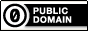Some epsilon-delta proofs

From Salas’s Calculus, 10th edition, page 104: Chapter 2 review exercise 45. Below, the important thing to keep in mind is that we want to use the “piecewise function idea”: that if a function can be thought of as a piecewise function, we first want to restrict it to where it is essentially nonpiecewise, and then show that the limit exists there.

Proof. We want to show $$\lim_{x\to-4} |2x+5| = 3$$. If $$|x+4|<1$$, then $$-1<x+4<1$$ so $$-2<2x+8<2$$ so $$-5<2x+5<-1$$, which means $$2x+5$$ is negative. But then $$|2x+5| = -(2x+5) = -2x -5$$. So we want to show that for all $$x$$ satisfying $$0<|x+4|<\delta$$, that $$\big||2x+5|-3\big|<\epsilon$$ holds. But using what we know, $$\big| |2x+5|-3 \big| <\epsilon$$ is the same thing as $$|-2x-5-3| = |-2x-8| = 2|x+4|<\epsilon$$ as long as $$|x+4|<1$$. So let $$\delta = \min\{1, \epsilon/2\}$$. Then $$|x+4|<\epsilon/2$$ so \begin{align*} -\epsilon/2 < x&+4 < \epsilon/2 \\ -\epsilon < 2x&+8 < \epsilon \\ -3 -\epsilon < 2x &+ 5 < -3 +\epsilon \ \ \ \ \ (\star) \\ \end{align*} But since $$|x+4|<1$$, we know \begin{align*} -1 < x&+4 < 1\\ -2 < 2x &+ 8 < 2 \\ -5 < 25 &+ 5 < -1 \end{align*} So $$2x+5 < 0$$ i.e. $$|2x+5| = -2x -5$$. From $$(\star)$$ we have then \begin{align} 3 + \epsilon > -2x - 5 > 3 - \epsilon \\ 3 + \epsilon > |2x +5| > 3-\epsilon\\ \epsilon > |2x+5|-3 > \epsilon \\ \big| |2x+5| -3\big| < \epsilon \ \ \ \ \ \square \end{align}

Tags: math.The content on this page is in the public domain.